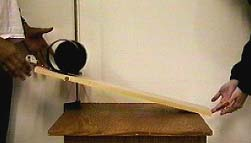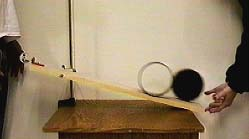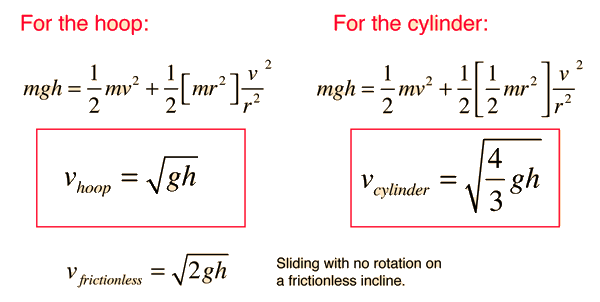Hoop and Cylinder Motion

Given a race between a thin hoop and a uniform cylinder down an incline. Which will win?Do the relative masses of the hoop and cylinder affect the outcome? Do the relative radii of the hoop and cylinder affect the outcome? Both start at the same height and have gravitational potential energy = mgh. Assume that they roll without slipping.

The analysis uses angular velocity and rotational kinetic energy. For rolling without slipping, the linear velocity and angular velocity are strictly proportional.
Index

Rotation concepts

Rotational kinetic energy concepts

 HyperPhysics***** Mechanics R Nave
Go Back

Hoop and Cylinder Motion

Given a race between a thin hoop and a uniform cylinder down an incline. Which will win?Conservation of energy gives:For rolling without slipping, ω = v/r. The difference between the hoop and the cylinder comes from their different rotational inertia.Solving for the velocity shows the cylinder to be the clear winner.The cylinder will reach the bottom of the incline with a speed that is 15% higher than the top speed of the hoop. The hoop uses up more of its energy budget in rotational kinetic energy because all of its mass is at the outer edge.

Index

Rotation concepts

Rotational kinetic energy concepts

 HyperPhysics***** Mechanics R Nave
Go Back

Hoop and Cylinder Motion

Given a race between a thin hoop and a uniform cylinder down an incline, rolling without slipping. Which will win?Mass and radius cancel out in the calculation, showing the final velocities to be independent of these two quantities.The cylinder wins the downhill race because it doesn't use up as much of its energy resource in rotational kinetic energy. All of the hoop's mass is at the maximum radius, contributing more to its moment of inertia. Since the angular velocity is proportional to the linear velocity, it has to put more of its energy into rotational kinetic energy.